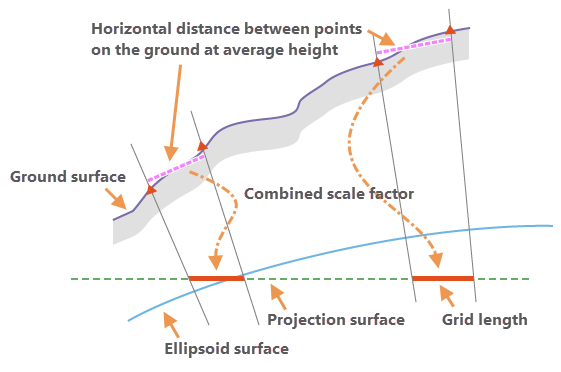# Ground to grid correction

Ground to grid correction is the process of converting ground measurements taken by surveyors or engineers to grid measurements on the projection surface of your map data. Setting accurate grid correction values ensures that new features are correctly rotated and scaled for a specific plane coordinate system.

When ground to grid correction is turned on, your input values are assumed to be ground measurements. An offset angle is applied to directions, and a distance factor multiplies distances. This occurs in real time as you create new geometry using construction tools and type bearing and distance values.

To learn more, see Turn ground to grid corrections on or off.

The following sections introduce the basic concepts of ground to grid correction as it applies to creating parcel data.

## Coordinate geometry

Surveyors use coordinate geometry (COGO) to record the measurements they take in the field for purposes of mapping parcel boundaries, utility easements, and the location of related physical features such as buildings or road centerlines.

COGO describes only straight lines, circular arcs, and spirals. Straight lines have a starting point, a direction, and a distance. Circular arcs have an arc length, a chord distance, a radius, a central angle, and a tangent length. Spirals describe transitions to and from circular curves or to a straight tangent, for example, to record the designed alignment for a roadway or railway.

### COGO-enabled line features

COGO-enabled line feature classes draw with COGO symbology and include a label expression that labels each line with its COGO dimension, when it exists. When you create new geometry using COGO-enabled construction tools, your input values are stored with the feature in COGO attribute fields.

## Direction offset

The direction offset is a user-defined angle that converts direction values to grid bearings on the projection surface of your map data. On survey documents, north can be geodetic north, magnetic north, grid north, or a locally defined north.

Surveyors are also free to choose a basis of bearing that is a line between two control points in the field as the baseline for a survey. You can find the basis of bearing or convergence angle as a statement on a survey document noting the two points used for the baseline. For example, Basis of Bearing is S 00'20'00" E 819.85.

## Distance factor

The distance factor is a user-defined multiplier that converts ground distances to grid distances as you create new geometry. Surveyors measure ground distances between two point locations on a level two-dimensional plane at an elevation above sea level that is effectively the ellipsoidal height.

Although the distance factor is computed at a single point location for a particular deed or subdivision, for most use cases it can be applied to all line distances within the same document, as the distance factor does not vary significantly within an area that is represented in a single document.

While there is a measurable difference between ellipsoidal heights and the height above sea level, this difference is mathematically insignificant when computing a distance factor for most land record data.

### Grid scale factor

The grid scale factor converts distances between the geodetic distances on the ellipsoid surface and the grid plane of the projection. This factor is computed from the x,y location of the point and the properties of the projection surface.

### Elevation factor

The elevation factor converts distances between the geodetic distances on the ellipsoid surface and the horizontal ground distances. This factor is computed from the z-elevation of the point.

### Combined scale factor

The applied distance factor value is calculated as a combined scale factor using grid scale and elevation factors. The combined scale factor is the product of multiplying the grid scale factor and the elevation factor.

These two scale factors correct the combined linear distortions caused by the curvature of the earth and the ground height of the measured distances above the ellipsoid surface with respect to the projection surface.